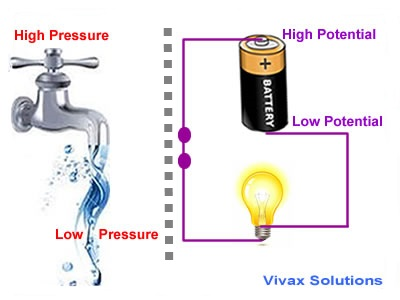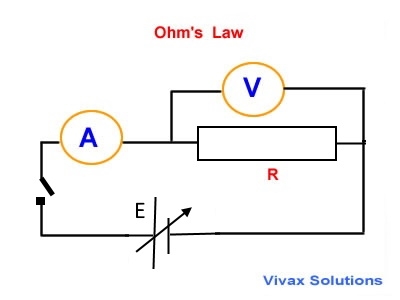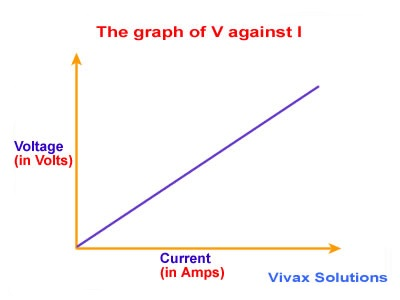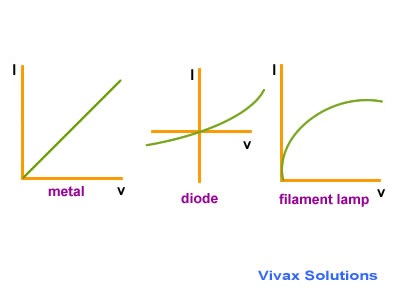### What makes a current flow in a circuit?When a tap opens, water flows out from the open end to the container below it. This is because of the fact that
water flows from a point of high pressure to a point of low pressure. In the same way, an electric current flows through a circuit from a point of high 'electric pressure' to a point of low 'electric pressure'.
In electricity, 'pressure' is known as potential. Therefore, an electric current flows through a circuit from a point of high potential to a point of low potential.
Since the positive terminal of a cell is at a higher potential than the negative terminal, an electric current goes from the positive terminal to the negative terminal via the circuit.
The flow of electrons, however, takes place in the opposite direction - from the negative to positive.
In other words, there should be a difference in potential between two points, if a current is to flow in a circuit. The potential difference is also known as voltage between the two points in question.

E.g.If two adjacent points in a circuit are connected to the earth, the potentials of the two points are the same; potential difference or voltage is zero.
Therefore, in theory, no current will flow through the points in the given scenario.

#### Potential Difference -voltage

The work done in moving a unit positive charge between two points in a circuit is called the potential difference or voltage between the two points.
V = W/Q
Units: J/C = Volts

E.g.1

Calculate the work done when 10000 electrons pass through a voltage of 12V. The charge of an electron is 1.6x10-19C.
W = QV
W = 10000 x 1.6x10-19 x 12 = 1.92x10-14J.

E.g.2

Calculate the voltage between two points, if the work done on 6C is 216J.
W = QV
216 = 6V
V = 36V.

E.g.3

It is highly unlikely that a bulb connected to the Earth terminal and the neutral wire on a wall socket would light up. Explain.
Since both ends are at zero potential, there is no potential difference between the two. So, there would be no flow of current through the bulb for it to light up.

E.g.4

Sarah complains that one of her wall sockets is not functioning properly. She checked the live and neutral terminals using a domestic electric tester. Both indicate the presence of electricity, but the appliance does not work. Give a possible explanation.
If the circuit is not faulty, the neutral wire should not indicate the presence of electricity in it. The live wire and the neutral may be in contact at some point in the circuit, which in turn keeps the two wires at the same potential.
Since there is no potential difference, the bulb will not work, when connected.

#### Ohm's Law

If the physical conditions remain constant, the voltage across a resistor is directly proportional to the current through it.

Please move the slider and experiment with it.

VI

V ∝ I
V = kI
k = R = resistance
V = IR

The physical conditions are temperature, pressure, humidity etc.

E.g.1

When a filament lamp is switched on, the most notable physical factor - the temperature - goes up. Is Ohm's law still valid in these circumstances for a light bulb?

Yes, only after the temperature of the filament becomes steady at some point.

E.g.2

The voltage across a resistor is 8V and the current through it is 2A. Find the resistance.
V = IR
8 = 2R
R = 4 Ω.

#### Investigating Ohm's LawChange the strength of the power source, a battery in this case, and measure the current through the ammeter and the voltage across the resistor. Then plot a graph of V against I.If the graph is a straight line that goes through the origin, it shows Ohm's Law is correct.

#### Ohmic Conductors

The substances that obey Ohm's Law are Ohmic conductors.

E.g. metals

#### Non-Ohmic Conductors

The substances that do not obey Ohm's Law are Non-Ohmic conductors.
E.g. semiconductor diodes, filament lampsThe I/V graph for Ohmic conductors is a straight line; for semiconductors and filament lamp, they are curvy.The best book for both teachers and students to learn physics - exactly like in the good old days:concepts are clearly explained in detail;no meaningless cartoons to devour space;the author rendered a great service in his unique approach for generations of students, with this being the fourth edition.

### GCSE Physics - Flash Cards

Amazon Best SellerPhysics flash cards have become an Amazon Best Seller; they are intuitive and summarizes the topic contents really well in beautiful layouts. They are vital for your forthcoming exams.

### A Level Physics - Flash CardsIt's not easy to find flash cards for A Level physics. These cards fill the void with lots of cards, covering the major topics that you need to know. The layout is beautiful and inspiring.